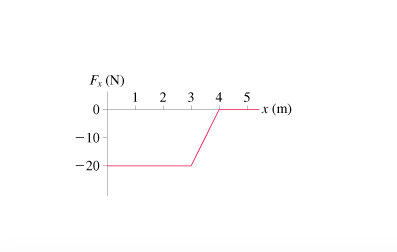1
0
watching
390
views

#A100 g particle experiences the one-dimensional, conservative force Fx shown in the figure(Figure 1). a)Let the zero of the potential energy be at x = 0 m.What is the potential energy at x = 1.0, 2.0, 3.0, and 4.0 m? Hint: Think about the definition of potential energy and the geometric interpretation of the work done by a varying force. Express your answers using two significant figures separated by commas. b)Suppose the particle is shot toward the right from x = 1.0 m with a speed of 19 m/s. Where is the particle's turning point?